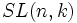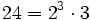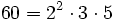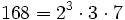# Special linear group

(diff) ← Older revision | Latest revision (diff) | Newer revision → (diff)
This article defines a natural number-parametrized system of algebraic matrix groups. In other words, for every field and every natural number, we get a matrix group defined by a system of algebraic equations. The definition may also generalize to arbitrary commutative unital rings, though the default usage of the term is over fields.
View other linear algebraic groups|View other affine algebraic groups

## Definition

### In terms of natural numbers

Let$n$ be a natural number and$k$ a field. The special linear group of degree$n$ over$k$ is defined in the following equivalent ways:

1. It is the group of all invertible matrices of order$n$, having determinant 1.
2. It is the kernel of the determinant homomorphism from the general linear group of degree$n$ over$k$ to the multiplicative group of$k$.

## More general versions

Replacement of field Most direct analogue of special linear group Other less direct analogues
Division ring special linear group over a division ring
Commutative unital ring special linear group over a commutative unital ring Steinberg group over a commutative unital ring, group generated by elementary matrices over a commutative unital ring

## As a map

### As a functor from fields to groups

If we fix$n$, we can think of$SL_n$ as a functor from the category of fields to the category of groups.

### As an IAPS

Further information: SL IAPS

For a fixed field$k$, the general linear groups$SL(n,k)$ form an IAPS of groups parametrized by$n$. In other words, we naturally have concatenation maps:$\Phi_{m,n}: SL(m,k) \times SL(n,k) \to SL(m+n,k)$

This map takes a matrix$A$ of order$m$ and a matrix$B$ of order$n$ and putputs the block diagonal matrix with blocks$A$ and$B$.

### As a functor from fields to IAPSes

If we fix neither$n$ nor$k$, we can view$SL$ as a functor from fields to the category of IAPSes of groups.

## Generating sets and presentations

### Generating sets for the special linear group

• Elementary matrices generate the special linear group: This is true for all special linear groups over all fields.
• Dickson's theorem: This shows that$SL(2,q)$ is practically always generated by any upper-triangular unipotent mtarix and any lower-triangular unipotent matrix.

### Presentations for the special linear group

• Steinberg presentation for the special linear group: This is a presentation that works for special linear groups over fields. Over rings that are not fields, the presentation gives a group that has a homomorphism to the special linear group that need not in general be either injective or surjective.

## Particular cases

### Finite fields

Here are some general facts:

• Special linear group is quasisimple for$n \ge 2$, except the case that$n = 2$ and$k$ has two or three elements. Thus, all the corresponding general linear groups have a simple non-abelian subquotient. In the case that the field has characteristic two, the general linear group coincides with the special linear group, and it is centerless, so it turns out to be a simple non-abelian group itself.
Size of field Order of matrices Common name for the special linear group Order of group Comment$q$ 1 Trivial group$1$ Trivial
2 2 Symmetric group:S3$6 = 2 \cdot 3$ supersolvable but not nilpotent
3 2 Special linear group:SL(2,3)$24 = 2^3 \cdot 3$ solvable but not supersolvable
4 2 Alternating group:A5$60 = 2^2 \cdot 3 \cdot 5$ simple non-abelian group
5 2 Special linear group:SL(2,5)$240 = 2^4 \cdot 3 \cdot 5$ quasisimple, with inner automorphism group isomorphic to alternating group:A5.
2 3 General linear group:GL(3,2)$168 = 2^3 \cdot 3 \cdot 7$ simple non-abelian group

## GAP implementation

The GAP command for constructing the special linear group is GAP:SpecialLinearGroup. It can be invoked using either SpecialLinearGroup or SL. It takes two arguments, the first of which is the degree (i.e., the order of matrices) and the second is either a ring or a prime power (for which the corresponding field is considered the ring). For instance, to define$SL(2,3)$, we write:

G := SL(2,3);